What does it do?

It returns the position of an item in a range

Formula breakdown:

=MATCH(lookup_value, lookup_array, [match_type])

What it means:

=MATCH(lookup this value, from this list or range of cells, return me the Exact Match)

The MATCH function in Excel returns the position of an item within a list or a range of cells.

Say that you have a Price List and want to know in which position a certain item is located within that Price List, then you would use the MATCH function.

NB: The lookup_value argument can be a value (number, text, or logical value) or a cell reference to a number, text, or logical value.

We want to get the position within the Stock list where the Tablet is located.

STEP 1: Enter the following:

We need to enter the MATCH function in a blank cell:

## =MATCH(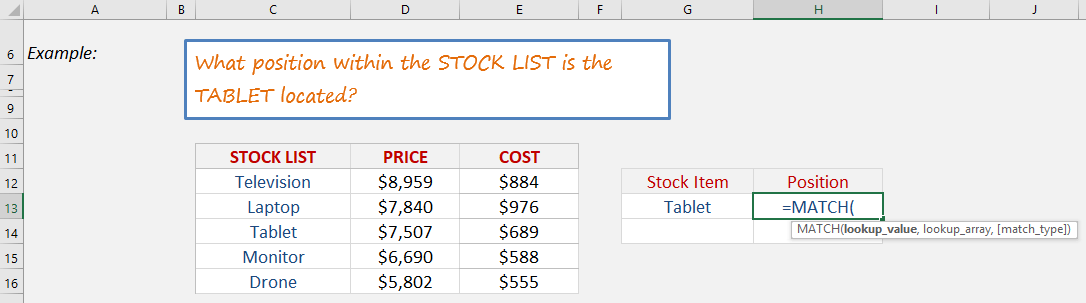STEP 2: The MATCH arguments:

## Lookup_value

What is the value that we want to match?

We want to match the Tablet.

## =MATCH(G13,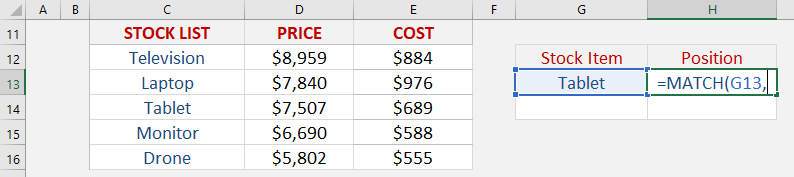## Lookup_array

Where is the list that contains the stock items?

## =MATCH(G13, C12:C16,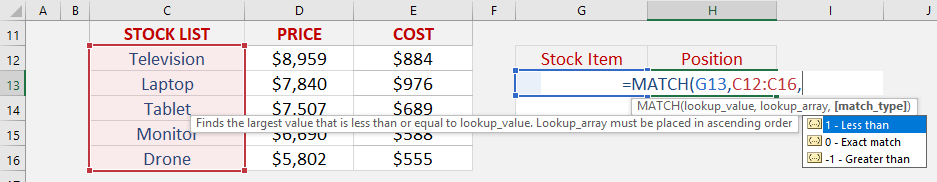## Match_type

What kind of matching do you want?

Let’s put in 0 to get the exact match

## =MATCH(G13, C12:C16, 0)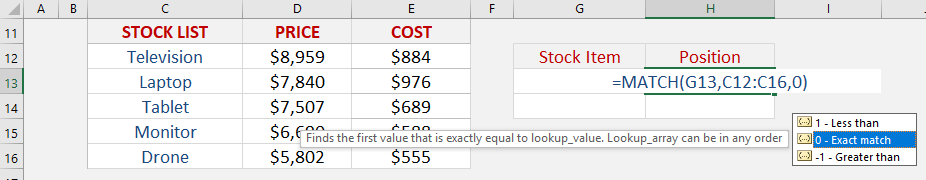And with that, you will get that tablet is in Row #3!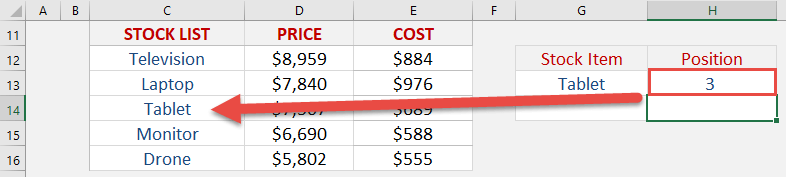How to Use the Match Formula in Excel